Ch 6. Entropy Multimedia Engineering Thermodynamics Entropy Tds Relations EntropyChange IsentropicProcess IsentropicEfficiency EntropyBalance (1) EntropyBalance (2) ReversibleWork
 Chapter 1. Basics 2. Pure Substances 3. First Law 4. Energy Analysis 5. Second Law 6. Entropy 7. Exergy Analysis 8. Gas Power Cyc 9. Brayton Cycle 10. Rankine Cycle Appendix Basic Math Units Thermo Tables Search eBooks Dynamics Fluids Math Mechanics Statics Thermodynamics Author(s): Meirong Huang Kurt Gramoll ©Kurt GramollTHERMODYNAMICS - THEORY Reversible Steady-flow Work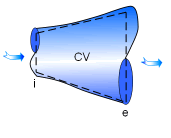One-inlet-one-exit Nozzle The energy balance for a steady-flow device (nozzle, compressor, turbine and pump) with one inlet and one exit is: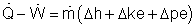Its differential form is:       δq - δw = dh + dke + dpe If the device undergoes an internally reversible process, the heat transfer term δq can be replaced by dh - vdP since       δqrev = Tds       Tds = dh - vdP (the second Tds relation) Then the energy balance becomes       dh - vdP - δwrev = dh + dke + dpe By rearranging the above equation, the reversible steady-flow work can be expressed as       - δwrev = vdP + dke + dpe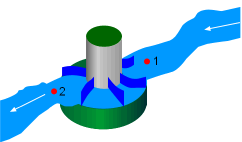Water Flowing through a Hydraulic Turbine Integrating it form location 1 to location 2 yields,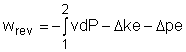The above equation is the relation for the reversible work output associated with an internally reversible process in a steady-flow device. When the changes in kinetic and potential energies are negligible, the relation reduces to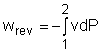The above equation states that the larger the specific volume, the larger the reversible work produced or consumed by a steady-flow device. To minimize the work input during a compression process, one should keep the specific volume of the working fluid as small as possible. In the same manner, to maximize the work output during an expansion process, one should keep the specific volume of the working fluid as large as possible.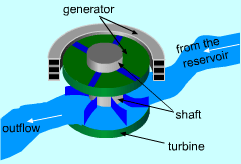Power Generated by Hydraulic Turbine One needs to know the relationship between the specific volume v and the pressure P for the given process to perform the integration in the above relation. If an incompressible fluid is used as the working fluid, the specific volume v is a constant. The relation for the reversible work output associated with an internally reversible process in a steady-flow device is simplified to give       wrev = -v(P2 - P1) - Δke - Δpe Hydraulic turbines used in hydroelectric power plants run in a steady-flow process with incompressible fluid, i.e., water, as the working fluid.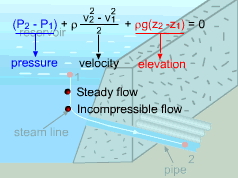The Bernoulli Equation If no work interactions are involved, like nozzle or pipe section, the above equation is reduced to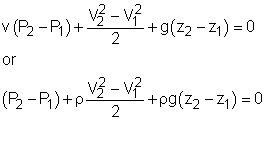where V is the velocity of the fluid. This equation is known as the Bernoulli equation in fluid mechanics. Reversible Steady-flow Devices produce Most and Consume Least Work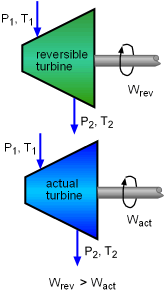Reversible Turbine Delivers more Work than Actual Turbine The steady-flow devices deliver the most and consume the least work when it undergoes a reversible process. Consider two steady-flow devices, one is reversible and the other is irreversible (actual process), operating between the same inlet and exit states. The differential forms for the energy balance of these two devices are       δqact - δwact = dh + dke + dpe       δqrev - δwrev = dh + dke + dpe The right hand sides of these two equations are the same. It gives,       qact - δwact = qrev - δwrev Rearranging this equation gives,       δwrev - δwact = qrev - qact Since qrev = Tds, the above equation becomes,       δwrev - δwact = Tds - qact the increase of entropy principle gives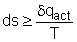Thus,       δwrev - δwact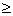0 or δwrevδwact That is, for the same inlet and exit conditions, when the device undergoes a reversible process, a work-produce device like turbine produces the most work (w is positive), or a work-consuming device like compressor consumes the least work (w is negative).